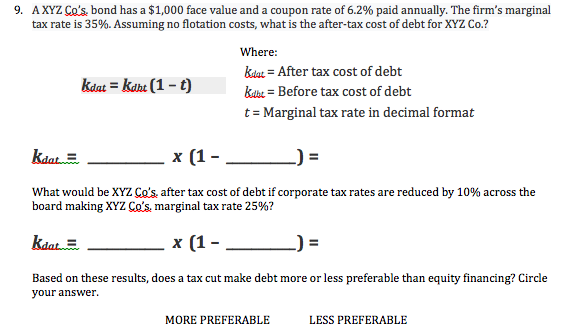# AXYZ Co's. bond has a $1,000 face value and a coupon rate of 6.2% paid annually. The firm's marginal tax rate is 35%. Assuming no flotation costs, what is the after-tax cost of debt for XYZ Co.? 9. Where kdtar =After tax cost of debt ka kahu (1-t) kabe = Before tax cost of debt t Marginal tax rate in decimal format x (1 kdat What would be XYZ Co's. after tax cost of debt if corporate tax rates are reduced by 10% across the board making XYZ Co's. marginal tax rate 25%? kdat x (1-_ Based on these results, does a tax cut make debt more or less preferable than equity financing? Circle your answer LESS PREFERABLE MORE PREFERABLE Questionhelp_outlineImage TranscriptioncloseAXYZ Co's. bond has a$1,000 face value and a coupon rate of 6.2% paid annually. The firm's marginal tax rate is 35%. Assuming no flotation costs, what is the after-tax cost of debt for XYZ Co.? 9. Where kdtar =After tax cost of debt ka kahu (1-t) kabe = Before tax cost of debt t Marginal tax rate in decimal format x (1 kdat What would be XYZ Co's. after tax cost of debt if corporate tax rates are reduced by 10% across the board making XYZ Co's. marginal tax rate 25%? kdat x (1-_ Based on these results, does a tax cut make debt more or less preferable than equity financing? Circle your answer LESS PREFERABLE MORE PREFERABLE fullscreen

## Expert Answer

### Want to see this answer and more?

Experts are waiting 24/7 to provide step-by-step solutions in as fast as 30 minutes!*

*Response times vary by subject and question complexity. Median response time is 34 minutes and may be longer for new subjects.
Tagged in© 2021 bartleby. All Rights Reserved.